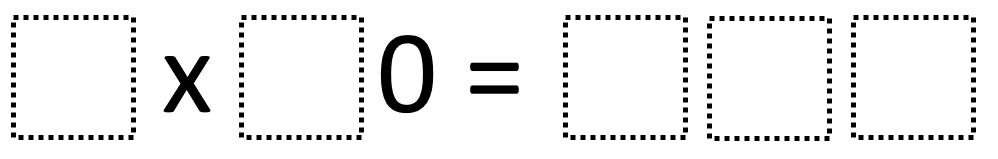# Multiplying Multiples Of Ten 2

Directions: Using the digits 0 to 9 at most one time each, place a digit in each box to make a product that’s as close to 500 as possible.### Hint

How can you tell where the zero will go? How can your knowledge of multiplying one-digit numbers help you here?

The closest you can get to 500 is 480 from multiplying 8 x 60 or 6 x 80.

Source: Robert Kaplinsky

## Multiply and Divide Within A Hundred 1

Directions: Using the digits 2 to 9 at most one time each, place a digit …

1.Marcie Longstaff

Doesn’t the Answer use the number 8 twice?

2.6 x 80=480

3.The answer given uses 8 twice. I think 9 x 60 = 540 or 6 x 90 = 540 may be the closest.

4.My students noticed that your answer suggestion uses the digit “8” twice.